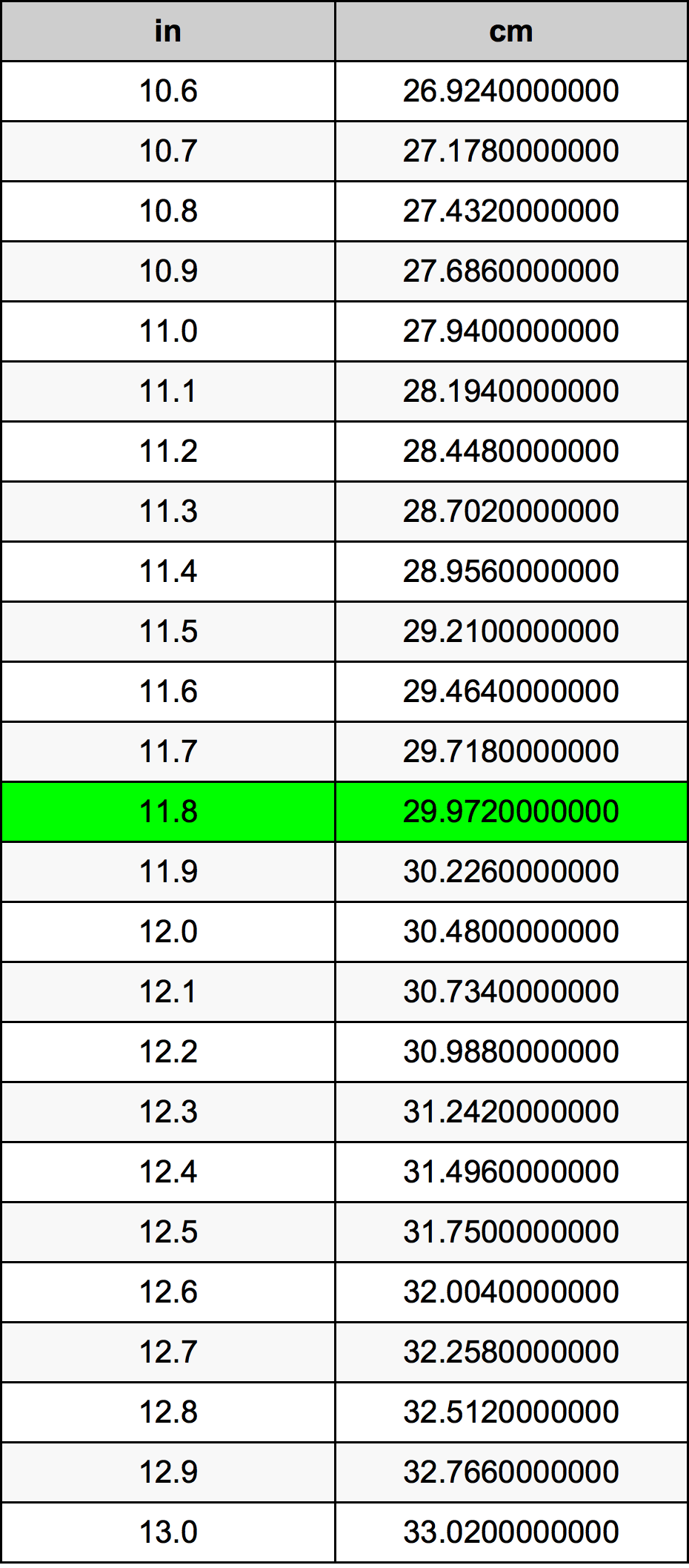Inches To Centimeters

# 11.8 in to cm11.8 Inches to Centimeters

in
=
cm

## How to convert 11.8 inches to centimeters?

 11.8 in * 2.54 cm = 29.972 cm 1 in
A common question is How many inch in 11.8 centimeter? And the answer is 4.6456692913 in in 11.8 cm. Likewise the question how many centimeter in 11.8 inch has the answer of 29.972 cm in 11.8 in.

## How much are 11.8 inches in centimeters?

11.8 inches equal 29.972 centimeters (11.8in = 29.972cm). Converting 11.8 in to cm is easy. Simply use our calculator above, or apply the formula to change the length 11.8 in to cm.

## Convert 11.8 in to common lengths

UnitLength
Nanometer299720000.0 nm
Micrometer299720.0 µm
Millimeter299.72 mm
Centimeter29.972 cm
Inch11.8 in
Foot0.9833333333 ft
Yard0.3277777778 yd
Meter0.29972 m
Kilometer0.00029972 km
Mile0.0001862374 mi
Nautical mile0.0001618359 nmi

## What is 11.8 inches in cm?

To convert 11.8 in to cm multiply the length in inches by 2.54. The 11.8 in in cm formula is [cm] = 11.8 * 2.54. Thus, for 11.8 inches in centimeter we get 29.972 cm.

## 11.8 Inch Conversion Table## Alternative spelling

11.8 Inch to Centimeters, 11.8 Inch in Centimeters, 11.8 Inch to cm, 11.8 Inch in cm, 11.8 in to Centimeter, 11.8 in in Centimeter, 11.8 Inches to Centimeters, 11.8 Inches in Centimeters, 11.8 in to Centimeters, 11.8 in in Centimeters, 11.8 Inches to Centimeter, 11.8 Inches in Centimeter, 11.8 in to cm, 11.8 in in cm